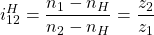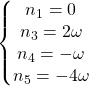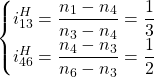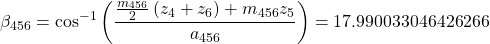# 基于傅里叶级数设计平面绘图机构

## 灵感来源

https://www.bilibili.com/video/BV1vt411N7Ti?share_source=copy_web

## 傅里叶级数相关理论1. 使用复函数形式表示图形（读取svg图像、自己手写）
2. 使用数值积分计算复系数
3. 将所有项相加

## 参数计算的MATLAB实现

keypoint = zeros(7,2);

keypoint(1,:) = [300 0];
keypoint(2,:) = [-300 1/6];
keypoint(3,:) = [-300*cos(pi/3)-300*sin(pi/3)*1i 2/6];
keypoint(4,:) = [300*cos(pi/3)+300*sin(pi/3)*1i 3/6];
keypoint(5,:) = [-300*cos(pi/3)+300*sin(pi/3)*1i 4/6];
keypoint(6,:) = [300*cos(pi/3)-300*sin(pi/3)*1i 5/6];
keypoint(7,:) = [300 1];

f = @(t) interp1(keypoint(:,2), keypoint(:,1), t);

t = 0:.001:1;
s = f(t);
s_real = real(s);
s_imag = imag(s);
plot(s_real, s_imag);
axis equal

c = complex(201, 1);
max_n = 100;
for n = -max_n:max_n
ef = @(t) exp(-2*pi*1i*n*t) .* f(t);
c(n+max_n+1) = integral(ef, 0, 1);
end

data = [];
m = 1;
for n = -max_n:max_n
if abs(c(n+max_n+1)) > 20
data(m, 1) = c(n+max_n+1);
data(m, 2) = n;
m = m + 1;
end
end

figure;
t = 0:.001:1;
s = f_appr(t, data(:,1), data(:,2));
s_real = real(s);
s_imag = imag(s);
plot(s_real, s_imag);
axis equal

function [ s ] = f_appr( t, c, n )
%f_appr 傅里叶级数

s = 0;
for m = 1:length(n)
s = s + c(m) .* exp(2*pi*1i*n(m)*t);
end

end


output = [abs(data(:,1)) angle(data(:,1))*180/pi data(:,2)];

## 机构设计

#### 基础单元#### 整体设计#### 机构平衡

• 机构静止时，机构自重会产生较大的倾覆力矩，这些力矩很大程度上由连杆的转轴承担
• 机构在运动时，由于每一级都会进行平面运动，并且每增加一级，角速度会成倍增加，故会产生严重的附加动载荷

• 由机构自重产生沿轴向的正压力（主要）
• 驱动轮系加减速、克服机构运动阻力的扭矩（次要）

## 总结

• 可绘制任意二维图形
• 图形精度可控
• 机构无冲击、无附加动载荷
• 零件复用程度高

• 机构平衡需要通过人工进行补偿
• 当选取级数增加时，机构尺寸成倍变小，造成设计困难
• 由于缺乏明确的应用场景，难以对机构各部件强度进行校核
• 目前配重块以串联形式叠加，当其数目较多时易发生弯曲变形

### 1人评论了“基于傅里叶级数设计平面绘图机构”

1.匿名

Very interesting and impressive work！